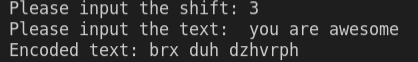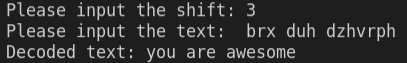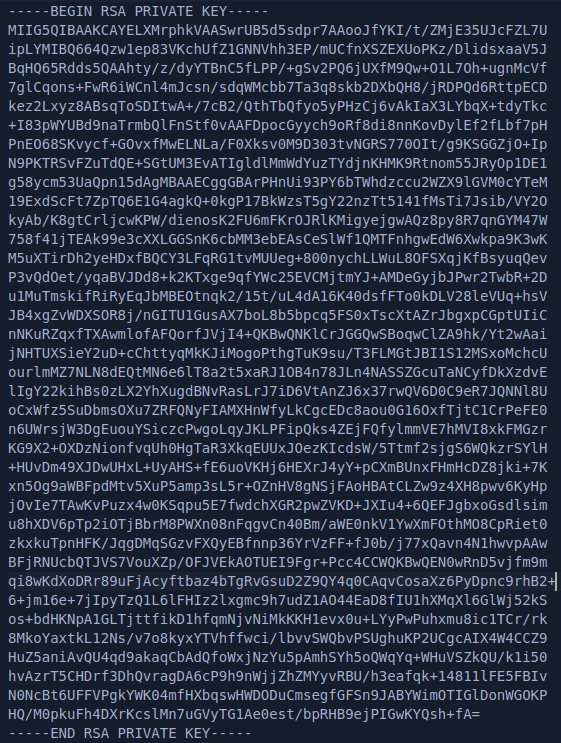Kiran Sethumadhavan I am a cyber security enthusiast who loves learning new technology that can keep the internet a secure place.

# Implementing cryptography with Python## What is cryptography?

Cryptography is the art of creating a secure communication channel by encrypting and decrypting data using code, meaning that no one other than the destined person can see the transmitted data.

Cryptography mainly uses mathematical principles and a series of formulas and algorithms to encrypt messages so that decrypting these messages becomes impossible. It’s used everywhere in today’s world, from securing day-to-day communication in social media platforms to securing banking transaction activity for online e-commerce.

## What are the different types of cryptography?

There are three primary types of cryptography:

1. Symmetric key cryptography
2. Asymmetric key cryptography
3. Hashing

Let’s look at each one in-depth!

## Symmetric key cryptography

Symmetric key cryptography is one of the fastest and easiest ways to decrypt and encrypt messages. It mainly involves using a single key, called a secret key, used for encrypting and decrypting the data. It’s also referred to as private-key cryptography, as well as secret-key, shared-key, one-key, and private-key encryption.

The data is converted into a form that is not understood by anyone, making the data secure from an attacker. It’s used primarily for encrypting large amounts of data. Symmetric key cryptography has two method types, which are used to convert plain text to ciphertext: block ciphers and stream ciphers.

Block ciphers use the algorithm mode of Electronic Code Block (ECB) and Cipher Code Block (CBC). These take text inputs and convert them into ciphertext by taking a block of text and generating a ciphertext of the same size. Block ciphers are relatively slower than stream ciphers, as a block of text needs to be covered, encrypted, and decrypted.

Stream ciphers, on the other hand, use Ciphertext Feedback (CFB) and Output Feedback (OFB) modes, which convert plain text into ciphertext by taking an input of 1 byte at once, making stream cipher faster than block cipher. Stream cipher uses XOR for converting plaintext into ciphertext.

Some examples of symmetric key algorithms are:

### Caesar cipher with Python

Caesar cipher is one example of symmetric key cryptography, and it’s one of the oldest and easiest ways to implement cryptography. Caesar cipher is a substitution cipher in which alphabets shift their order by a fixed number of positions.

Encrypting and decrypting Caesar cipher is easy, as the method is fixed and no key is required. Thus, anyone who knows the method will be able to decrypt the message easily.

For example, a simple word like “cat,” will be encrypted as “fdw,” if you shift each letter three letters in the alphabet. This makes the word “cat” difficult to understand if you’re unaware of the way it was encrypted.

Now, let’s create a simple Python program for encrypting and decrypting cipher text:

```alphabets = 'abcdefghijklmnopqrstuvwxyz'
def encrypt_caesar(num, text):
result = ' '
for k in text.lower():
try:
i = (alphabets.index(k) + num) % 26
results += alphabets[i]
except ValueError:
results+= k
return results.lower()
ciphertext = encrypt_caesar(num, text)
print(“Encoded text:”,ciphertext)```

Here’s the result:First, we created a variable named `alphabets` in which we have written all the alphabet letters. Then, we created a function named `encrypt_ceaser(num, text)` in which we will put the shift key and the text that has to be encrypted.

`for k in text.lower():`

By using a `for` loop, we would insert the input string in lowercase.

```for k in text.lower():
try:
i = (alphabets.index(k) - num) % 26
results += key[i]
except ValueError:
results += k
return results.lower()```

The `try` method and `except` method, `ValueError`, is used to catch errors between the program. After `try`, we count the letters in the string using `index`.

All of the alphabets in the text are added with the shift key and then divided by 26. Once the loop is complete, the letters are shifted by the shift value.

```num =int(input("Please input the shift:\t"))
ciphertext = decrypt_caesar(num, text)
print ("Decoded text:",ciphertext)
```

With the `input()` method, we take user input for the program. Then, a variable is created in ciphertext, which we call `encrypt_caesar( num, text)`. The `print` statement is used to print the encoded ciphertext.

Now, to decrypt the text, we will subtract by the same key value: `3`

```alphabets= 'abcdefghijklmnopqrstuvwxyz'
def decrypt_caesar(num, text):
results = ''
for k in text.lower():
try:
i = (alphabets.index(k) - num) % 26
results +=alphabets[i]
except ValueError:
results += k
return results.lower()
ciphertext = decrypt_caesar(num, text)
print(“Decoded text:”,ciphertext)```

Here’s the result of the decrypted text.Although this program is very similar to the previous program we created, we’ve made a few minor changes to decrypt the text.

Instead of using `i = (alphabets.index(k) + num)` % 26 we used a `-` in `i = (alphabets.index(k) - num) % 26` which decodes the ciphertext.

## Asymmetric key cryptography

Asymmetric key encryption is more complex and slower than symmetric cryptography. Also known as public-key cryptography, it involves using two different keys (and sometimes more) for encrypting and decrypting the data.

Essentially, a public key will be used to encrypt data, and only a corresponding private key will be able to decrypt the data, making the asymmetric key more secure.

### More great articles from LogRocket:

For example, you’re using asymmetric cryptography right now while reading this blog post. The lock symbol near your HTTPS website URL indicates that you are connected to this website securely using SSL/TLS certificates.

Asymmetric key encryption verifies the identity of the server and creates asymmetric encryption.

Some examples of asymmetric key algorithms are:

• Digital Signature Algorithm (DSA)
• Elliptic-curve cryptography (ECC)

Let’s generate an RSA key with Python using a Python package called Cryptodome:

```from Crypto.PublicKey import RSA
key = RSA.generate(3072)
file= open('Rsakey.pem','wb')
file.write(key.exportKey('PEM'))
file.close()```

Let’s install Cryptodome:

`pip install cryptodome`

To generate a key, we would use `key.generate(bit size)`. The `bit size` must be between 2048-4096 to make the key secure and lengthy.

`file = open('Rsakey.pem','wb')`

Here, `wb` **means to “write in binary.”

Next, let’s create a `.pem` (Privacy-Enhanced Mail), a file format used for storing cryptographic keys and certificates

`file.write(key.exportkey('PEM'))`

We will use the `.write` function to print the key in the file and use `.exportkey` to export the file in PEM format. Finally, we’ll close the file by using `file.close`.

Open the `Rsakey.pem file`:## Hashing

Hashing is the process of converting input of any length into a fixed-size string of text using mathematical algorithms. This means that any size of text, no matter how long it is, can be converted into an array of numbers and alphabets by an algorithm.

The mathematical algorithm used to convert the text is called a hash function, and the output is called a hash value. Hash algorithms are designed to be irreversible, making them secure from an attacker.

Hashing is used for user authentication and storing passwords. MD5 and SHA-1 hashing were used earlier for authentication and storing passwords, but they have since deprecated because they are insecure. Currently, the most secure algorithms are Argon2, bycrpt and scrypt, and PBKDF2.

Now, let’s create a simple hashing program so we can encrypt a string. First, let’s see how many hashing algorithms are available in Python by coding the following:

```import hashlib
Hash_Algorithms_available = hashlib.algorithms_available
print(Hash_Algorithms_available)

Output: {'sha256', 'md4', 'whirlpool', 'sha384', 'sha224', 'sm3', 'blake2s', 'blake2b', 'ripemd160', 'sha512_256', 'md5', 'sha512_224', 'shake_128', 'sha512', 'sha1', 'sha3_384', 'sha3_256', 'sha3_512', 'md5-sha1', 'shake_256', 'sha3_224'}```

First, let’s see a simple MD5 Python hashing program in action, as it’s one of the most common Python hashing examples:

```import hashlib #importing libraries
module = hashlib.md5() #selecting the hashing module
module.update(b"You are Awesome ") #inputting the text and b implies bytecode
print(module.hexdigest())```

The generated hex is:

`83d38079ecb05b2b0ad97ffa50a951eb`

Here, we import `hashlib`, a module available in Python, and create a variable named `module`, where we select the Python hashing algorithm to be used while hashing.

`.update` is an inbuilt function in hashlib that takes data as input and generates the hashes. The letter `b` indicates the string is a byte string, and `.digest` gives you the hash string generated from the data:

Now, let’s see a simple bcrypt Python hashing program in action.

```import bcrypt #importing libraries
input_password = b"YouareAwesome!" #inputting the text and b implies bytecode

The generated hex is: `b'\$2b\$12\$ZVMHgLah4CtGM1FGIXeEWusNA23wz1dqEc27a3rwwm9Fa4XVPLVLG'</code`

Bcrypt is a package available in Python that can be installed by a simple pip statement:

`pip install bcrypt`

We can then import the package `import bcrypt` and use the `bcrypt.hashpw()` function, which takes two arguments: `byte` and `salt`.

`Salt` is random data used in the hashing function that creates random strings and makes each hash unpredictable.

## Conclusion

In this article, you learned about cryptography and the various ways in which to encrypt data. We also created a simple Python program for encryption and decryption.

Implementing cryptography is extremely important. When you’re storing passwords in a database, make sure you are using the latest and strongest cryptography and hashing standards.Kiran Sethumadhavan I am a cyber security enthusiast who loves learning new technology that can keep the internet a secure place.